# Ohm's Law

In 1828, Georg Simon Ohm, a German physicist, derived a relationship between electric current and potential difference. This relationship is known as Ohm’s law. Certain formulas in Physics are so prevalent that they become popular knowledge that you end up memorising without trying. In the field of Modern Physics, it is E=m⋅c2. Similarly, in the field of current electricity, it is the Ohm’s law about which we will be studying in this article.

## Discovery of Ohm’s Law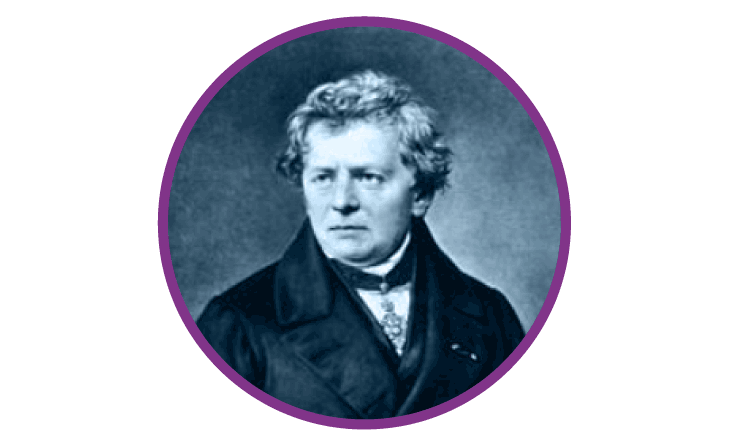Georg Simon Ohm was born in 1787, in Erlangen, Germany and taught mathematics in local schools and performed experiments in physics in a school physics laboratory, trying to master the principles behind electromagnetism. Later, in 1826, he published papers on the way circuits conducted heat in Fourier’s studies. In May 1827, Ohm published Die galvanische Kette, mathematisch bearbeitet, which described the relationship between electromotive force, current, and resistance later known as Ohm’s law. Having recieved muted response upon its initial release, Ohm’s findings would catalyze new research into electricity in the coming decades. Ohm was awarded the Royal Society’s highest award, the Copley Medal. Gradually, the term Ohm was adopted as the unit of electrical resistance in 1872.

## What is Ohm’s Law?

Ohm’s Law states that the current flowing through a conductor is directly proportional to the potential difference applied across its ends, provided the temperature and other physical conditions remain unchanged. Mathematically it can be represented as,

 Potential difference ∝ Current V ∝ I

( When the value of V increases the value of I increases simultaneously)

 V = IR

Where,

• V is Voltage in volts (V)
• R is Resistance in ohm (Ω)
• I is Current in Ampere (A)### How does Ohm’s Law work?

Ohm’s Law describes the current flow through a resistance when different electric potentials (voltage) are applied at each end of the resistance. Since we can’t see electrons, the model or an analogy of electric circuits used to help us understand circuits better is the water-pipe analogy. Water flowing through pipes is a good mechanical system that is analogous to an electrical circuit.

Here, the voltage is analogous to water pressure, the current is the amount of water flowing through the pipe, and the resistance is the size of the pipe. More water will flow through the pipe (current) when more pressure is applied (voltage) and the bigger the pipe, (lower the resistance).

### Different Applications of Ohm’s Law

The main applications of Ohm’s law are:

1. To determine the voltage, resistance or current of an electric circuit.
2. Ohm’s law is used to maintain the desired voltage drop across the electronic components.
3. Ohm’s law is also used in dc ammeter and other dc shunts to divert the current.

### Limitations of Ohm’s Law

Following are the limitations of Ohm’s law:

1. Ohm’s law is not applicable for unilateral electrical elements like diodes and transistors as they allow the current to flow through in one direction only.
2. For non-linear electrical elements with parameters like capacitance, resistance etc the voltage and current won’t be constant with respect to time making it difficult to use Ohm’s law.

## Calculating Different Parameters Using Ohm’s Law

As an equation, the Ohm’s Law serves as an algebraic recipe for calculating the current when the resistance and the potential difference are known. Likewise, if any two parameters in the equation are known, then the unknown third parameter can be easily calculated as follows:

• To find Voltage(V),
 V = IR
• To find Current(I),
 $I=\frac{V}{R}$
• To find Resistance(R),
 $R=\frac{V}{I}$

## Analyzing Simple Circuits Using Ohm’s Law

Resistive Circuits are analyzed using the Ohm’s Law. The law is often used to find the voltage, current or resistances of the circuit. The law relates these three quantities by the equation V = IR. Ohm’s Law Triangle helps us remember this equation while solving problems. Here, the three quantities V, I and R are superimposed into a triangle with voltage at the top and current and resistance below. The magic V I R  triangle can be used to calculate all formulations of Ohm’s law.

### The Ohms law Triangle

• If the value of voltage is asked and the values of the current and resistance are given, then to calculate voltage simply cover V at the top. So, we are left with the I and R orI X R. So, the equation for Voltage is Current multiplied by Resistance. Examples of how the magic triangle is employed to determine the voltage using Ohm’s law is given below.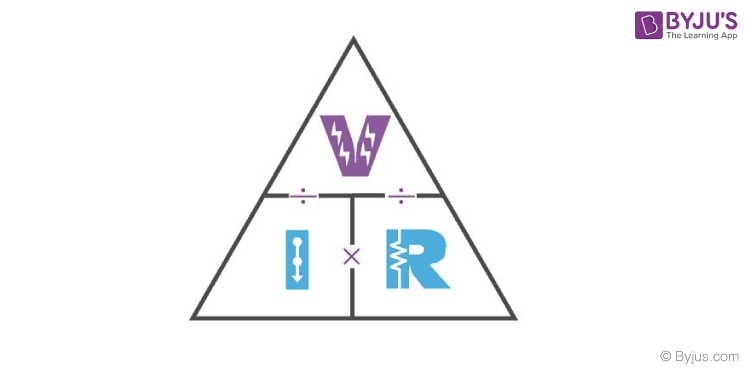## Solving Problems Using Ohm’s Law Triangle

Example 1: If the resistance of an electric iron is 50Ω and 3.2A Current flows through the resistance. Find the voltage between two points.

Ans.

If the value of Resistance is asked and the values of the current and voltage are given, then to calculate resistance simply cover the R. Now, we are left with the V at the top and I to the bottom left or V ÷ I.

Given, Resistance (R) = 50Ω
Current (I) = 3.2A
Therefore,
Voltage (V) = I X R = 3.2A x 50 Ω =160V

Example 2: An EMF source of 8.0 V is connected to a purely resistive electrical appliance (a light bulb). An electric current of 2.0 A flows through it. Consider the conducting wires to be resistance-free. Calculate the resistance offered by the electrical appliance.

Ans.
If the value of current is asked and the values of the resistance and voltage are given, then to calculate current simply cover the I. We are left with Voltage over Resistance or V ÷ R. So the equation for Current is Voltage divided by Resistance.
Given,
Voltage (V) = 8.0 V
Current (I) = 2.0 A

Therefore,
Resistance (R) = V ÷ I = $\frac{V}{I}$

= $\frac{8}{2} = 4 Ω$

## Calculating Electrical Power

The rate at which energy is converted from the electrical energy of the moving charges to some other form of energy like mechanical energy, heat, magnetic fields or energy stored in electric fields, is known as electric power. The unit of power is watt. The electrical power can be calculated using the Ohm’s law and by substituting the values of voltage, current and resistance.

### Formulae to find power

• When the values for voltage and current are given,
P = V x I
• When the values for voltage and resistance are given,
P = $V^{2}\div R$
• When the values for voltage and current are given,
P = $I^{2}\times R$

## What is Power Triangle?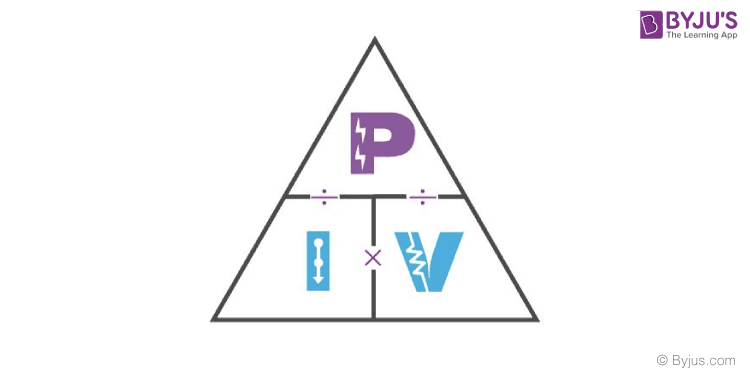In the power triangle, the power (P) will be on the top and current(I) and voltage (V) at the bottom.

• When the values of current and voltage will be given, the formula for finding power will be,
 P = I x V
• When the values of power and voltage will be given, the formula for finding current will be,
 I = $\frac{P}{V}$
• When the values of power and current will be given, the formula for finding voltage will be,
 V = $\frac{P}{I}$

## Ohm’s Law Pie Chart and Ohm’s Law Matrix Table

To better understand the relationship between various parameters, we can take all the equations used to find the voltage, current, resistance and power, and condense them into a simple Ohm’s Law pie chart as shown below.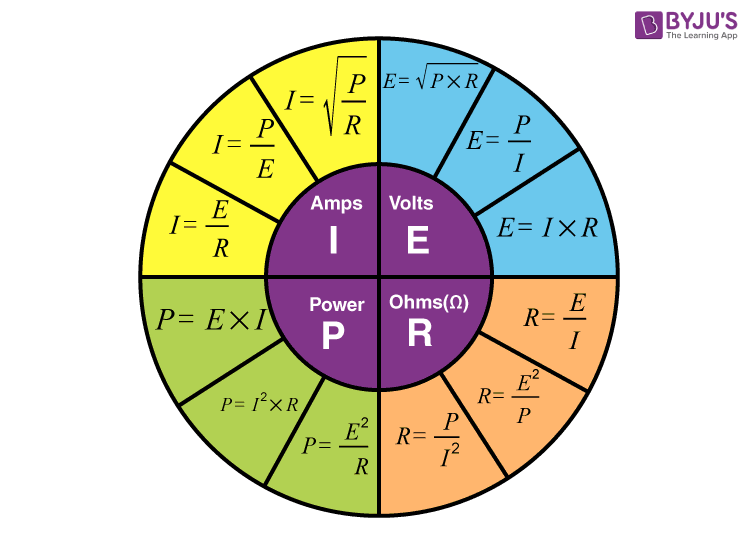Like Ohm’s Law Pie Chart shown above, we can condense the individual Ohm’s Law equations into a simple matrix table as shown below for easy reference when calculating an unknown value.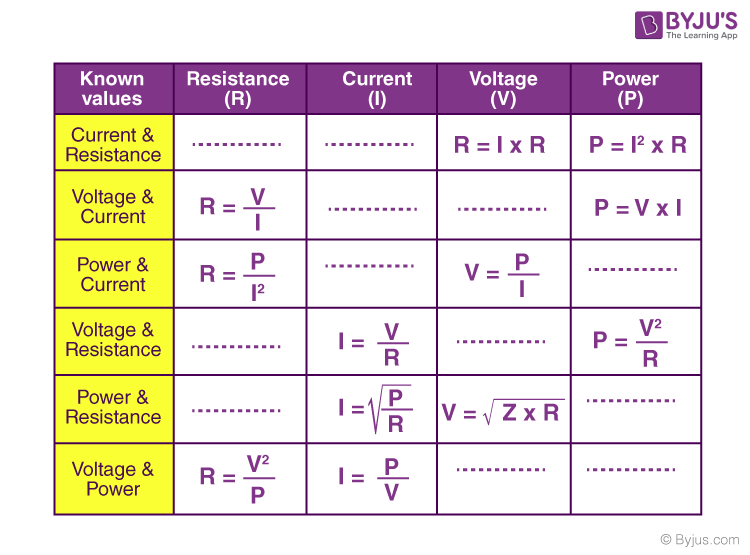### What is Ohm’s law simplified?

Ohm’s law states that the current passing through a conductor is proportional to the voltage over the resistance.

### How do you calculate current draw?

To calculate the current drawn, divide the power by the operating voltage.

### Do all metals obey Ohm’s law?

When the temperature of a metal increases, resistance decreases. Good conductors possess non-zero electrical resistances.

### Why is Ohm’s law important?

Ohm’s law formula is used to calculate electrical values so that we can design circuits and use electricity in a useful manner.

1. Venkatesh

Nice app to a students

Std nice this app

3. abdullah

thanks sir

4. Tayerera kudzai

Nice app for us

5. Ramita

Very good